Скачать презентацию A Model-Driven Approach for Generating Embedded Robot Navigation

947073bd233a48186c630ca805f03fe9.ppt

• Количество слайдов: 17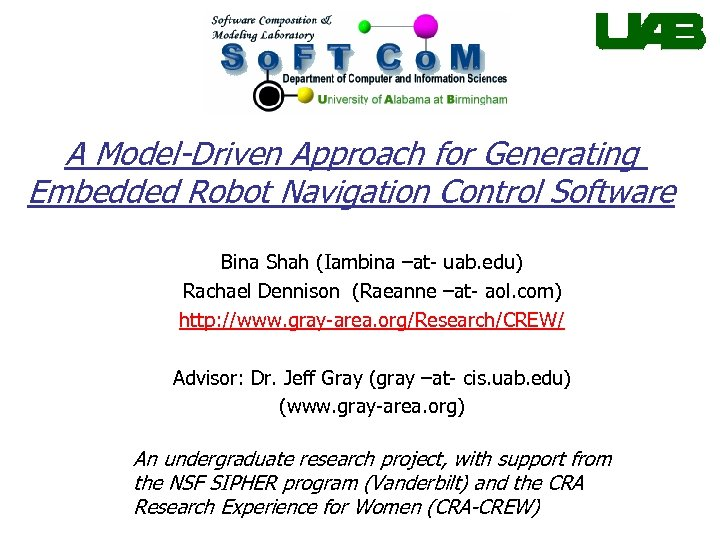A Model-Driven Approach for Generating Embedded Robot Navigation Control Software Bina Shah (Iambina –at- uab. edu) Rachael Dennison (Raeanne –at- aol. com) http: //www. gray-area. org/Research/CREW/ Advisor: Dr. Jeff Gray (gray –at- cis. uab. edu) (www. gray-area. org) An undergraduate research project, with support from the NSF SIPHER program (Vanderbilt) and the CRA Research Experience for Women (CRA-CREW)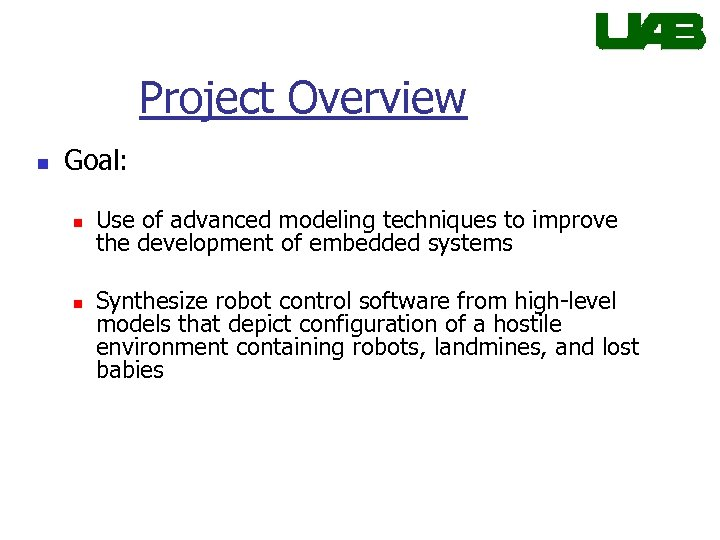Project Overview n Goal: n n Use of advanced modeling techniques to improve the development of embedded systems Synthesize robot control software from high-level models that depict configuration of a hostile environment containing robots, landmines, and lost babies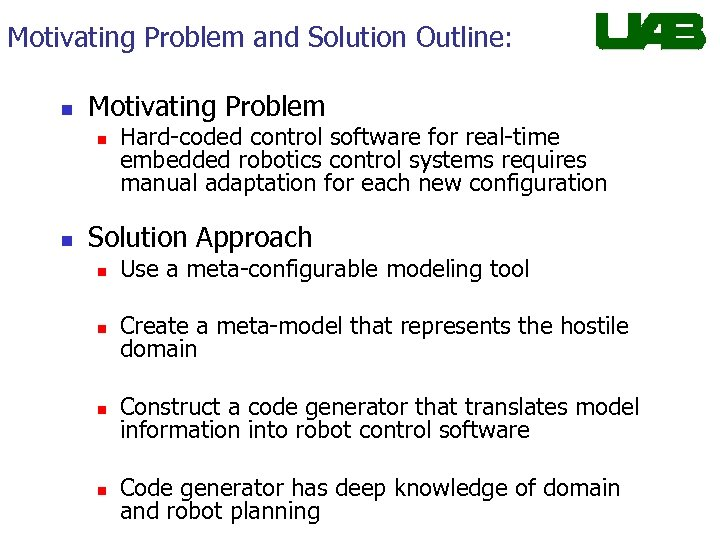Motivating Problem and Solution Outline: n Motivating Problem n n Hard-coded control software for real-time embedded robotics control systems requires manual adaptation for each new configuration Solution Approach n Use a meta-configurable modeling tool n Create a meta-model that represents the hostile domain n Construct a code generator that translates model information into robot control software n Code generator has deep knowledge of domain and robot planning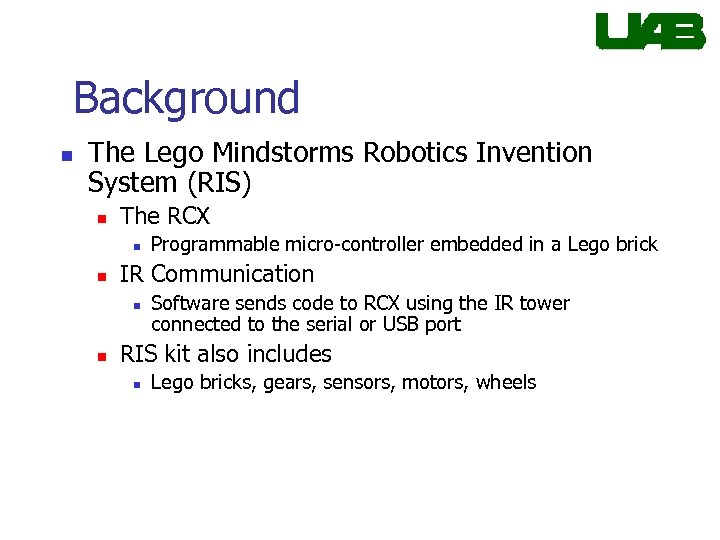Background n The Lego Mindstorms Robotics Invention System (RIS) n The RCX n n IR Communication n n Programmable micro-controller embedded in a Lego brick Software sends code to RCX using the IR tower connected to the serial or USB port RIS kit also includes n Lego bricks, gears, sensors, motors, wheels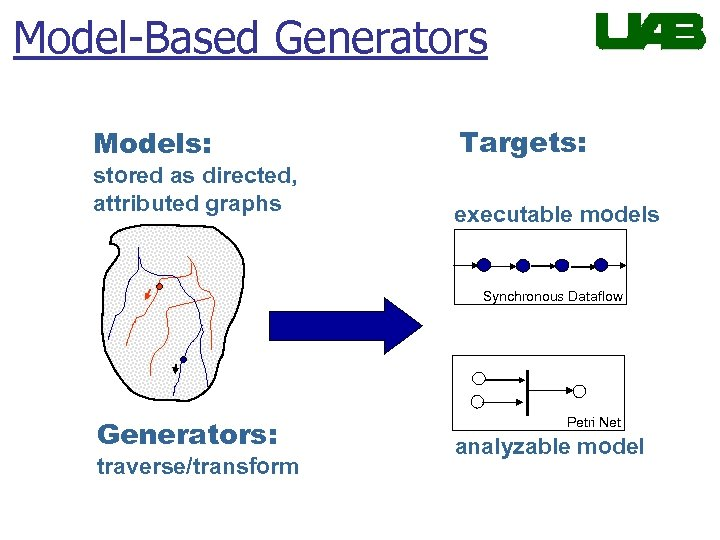Model-Based Generators Models: stored as directed, attributed graphs Targets: executable models Synchronous Dataflow Generators: traverse/transform Petri Net analyzable model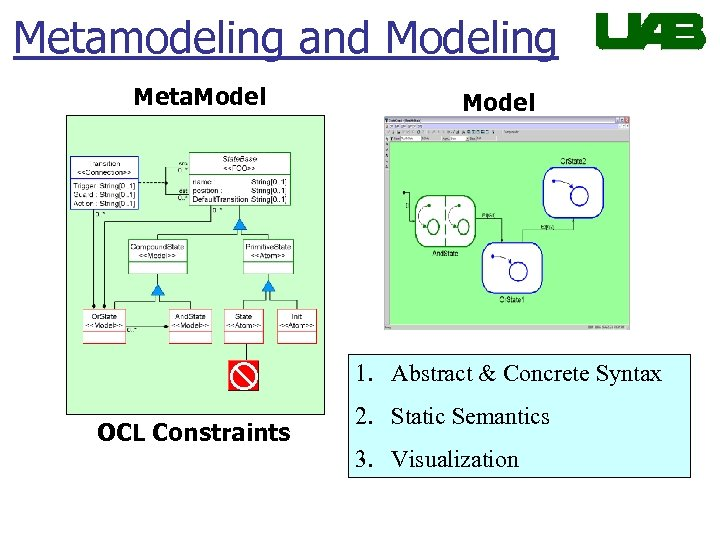Metamodeling and Modeling Meta. Model 1. Abstract & Concrete Syntax OCL Constraints 2. Static Semantics 3. Visualization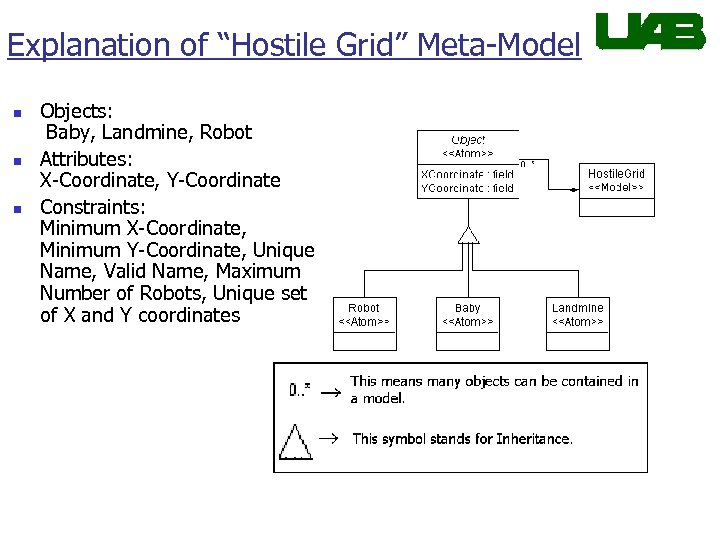Explanation of “Hostile Grid” Meta-Model n n n Objects: Baby, Landmine, Robot Attributes: X-Coordinate, Y-Coordinate Constraints: Minimum X-Coordinate, Minimum Y-Coordinate, Unique Name, Valid Name, Maximum Number of Robots, Unique set of X and Y coordinates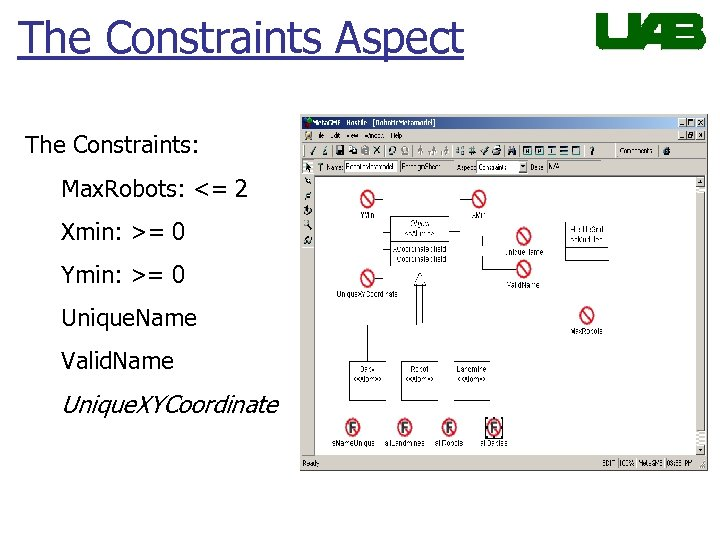The Constraints Aspect The Constraints: Max. Robots: <= 2 Xmin: >= 0 Ymin: >= 0 Unique. Name Valid. Name Unique. XYCoordinate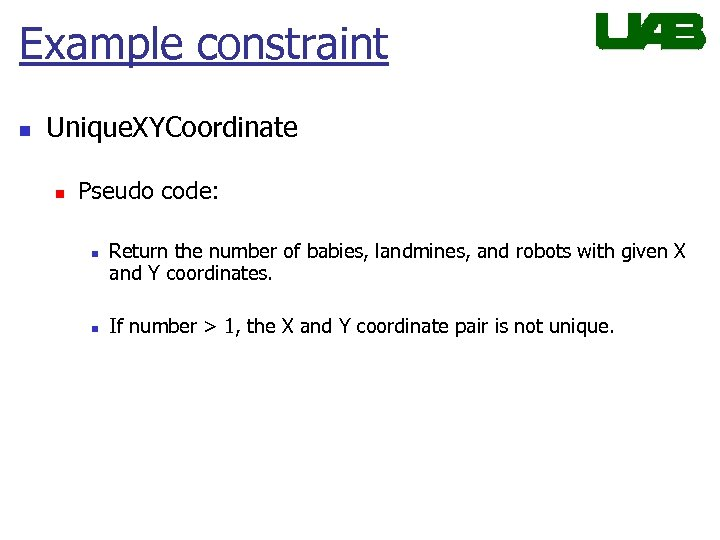Example constraint n Unique. XYCoordinate n Pseudo code: n n Return the number of babies, landmines, and robots with given X and Y coordinates. If number > 1, the X and Y coordinate pair is not unique.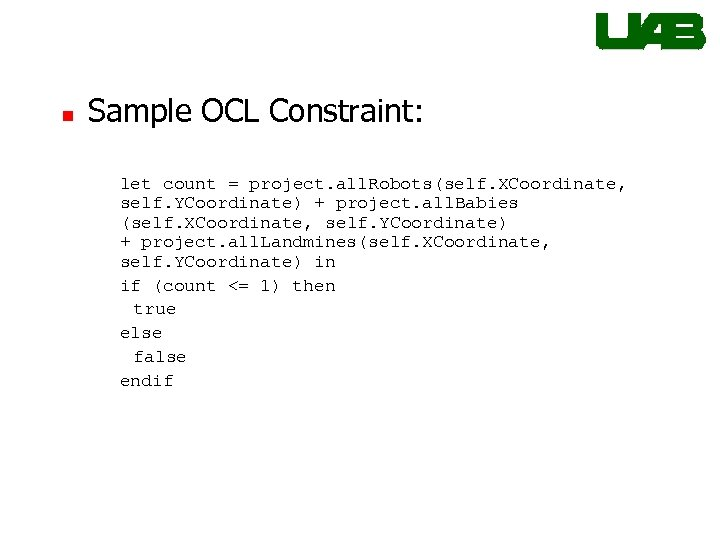n Sample OCL Constraint: let count = project. all. Robots(self. XCoordinate, self. YCoordinate) + project. all. Babies (self. XCoordinate, self. YCoordinate) + project. all. Landmines(self. XCoordinate, self. YCoordinate) in if (count <= 1) then true else false endif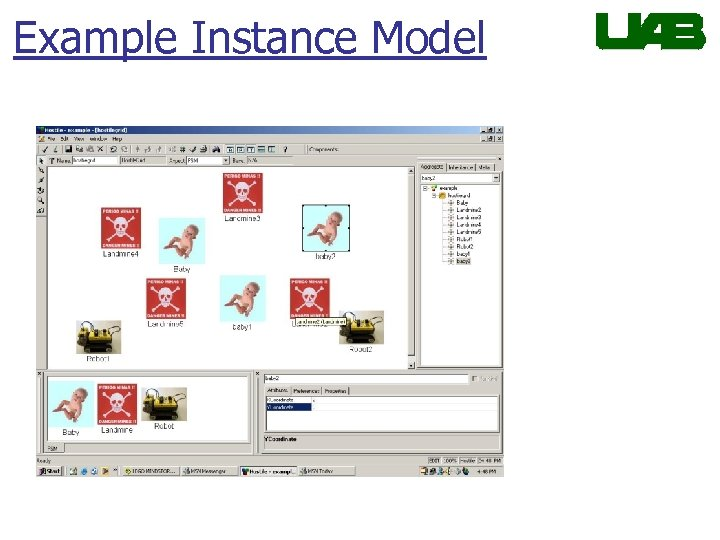Example Instance Model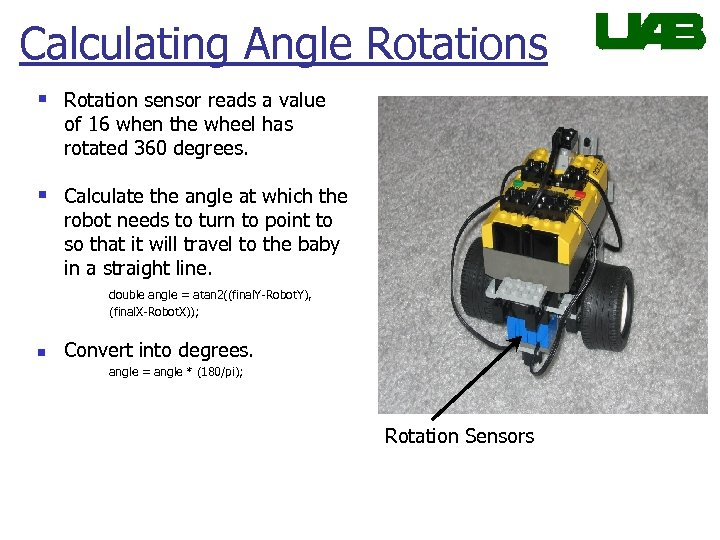Calculating Angle Rotations § Rotation sensor reads a value of 16 when the wheel has rotated 360 degrees. § Calculate the angle at which the robot needs to turn to point to so that it will travel to the baby in a straight line. double angle = atan 2((final. Y-Robot. Y), (final. X-Robot. X)); n Convert into degrees. angle = angle * (180/pi); Rotation Sensors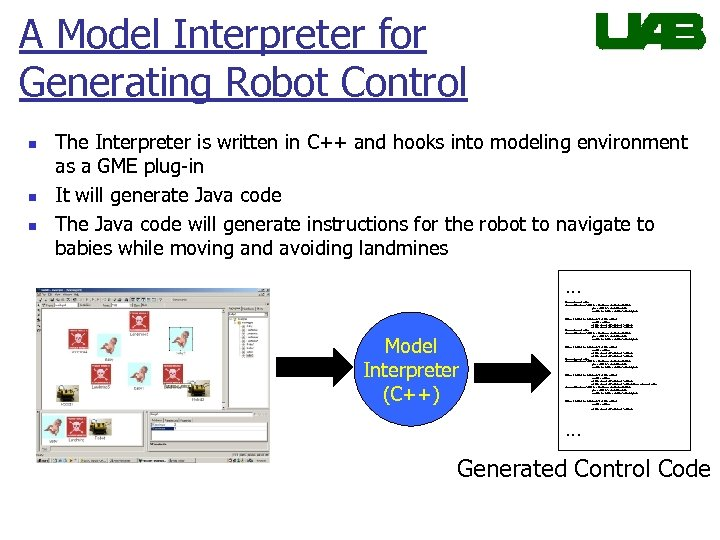A Model Interpreter for Generating Robot Control n n n The Interpreter is written in C++ and hooks into modeling environment as a GME plug-in It will generate Java code The Java code will generate instructions for the robot to navigate to babies while moving and avoiding landmines … //Get the hostile. Grid model const CBuilder. Atom. List *all. Robots = hostile. Diagram->Get. Atoms("Robot"); pos 1 = all. Robots->Get. Head. Position(); CBuilder. Atom *Robot = all. Robots->Get. Next(pos 1); Model Interpreter (C++) //obtain the robot's (X, Y) coordinates--> (Robot. X, Robot. Y) int Robot. X, Robot. Y; Robot->Get. Attribute("XCoordinate", Robot. X); Robot->Get. Attribute("YCoordinate", Robot. Y); //Get the hostile. Grid model const CBuilder. Atom. List *all. Robots = hostile. Diagram->Get. Atoms("Robot"); pos 1 = all. Robots->Get. Head. Position(); CBuilder. Atom *Robot = all. Robots->Get. Next(pos 1); //obtain the robot's (X, Y) coordinates--> (Robot. X, Robot. Y) int Robot. X, Robot. Y; Robot->Get. Attribute("YCoordinate", Robot. Y); … Generated Control Code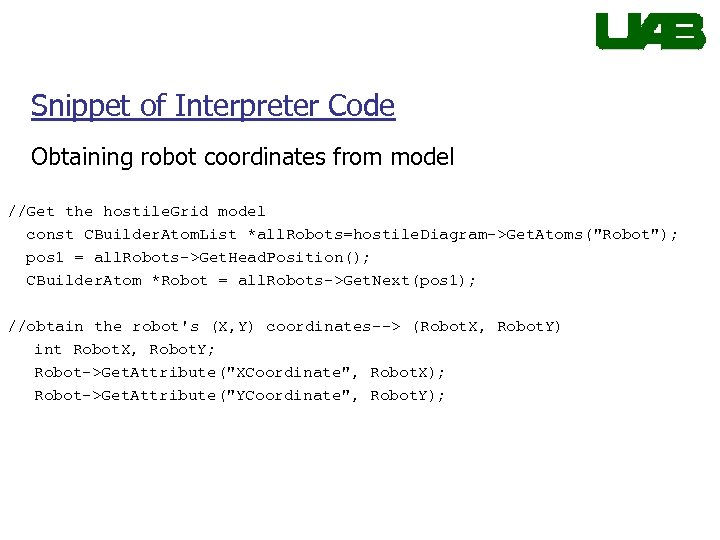Snippet of Interpreter Code Obtaining robot coordinates from model //Get the hostile. Grid model const CBuilder. Atom. List *all. Robots=hostile. Diagram->Get. Atoms("Robot"); pos 1 = all. Robots->Get. Head. Position(); CBuilder. Atom *Robot = all. Robots->Get. Next(pos 1); //obtain the robot's (X, Y) coordinates--> (Robot. X, Robot. Y) int Robot. X, Robot. Y; Robot->Get. Attribute("XCoordinate", Robot. X); Robot->Get. Attribute("YCoordinate", Robot. Y);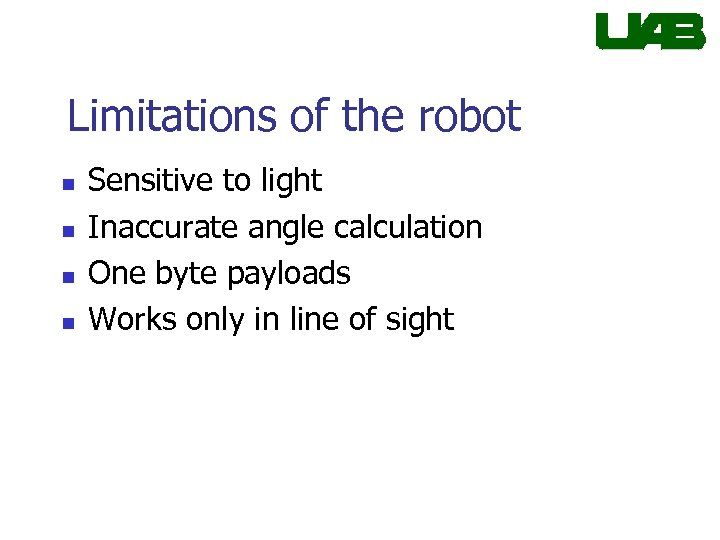Limitations of the robot n n Sensitive to light Inaccurate angle calculation One byte payloads Works only in line of sight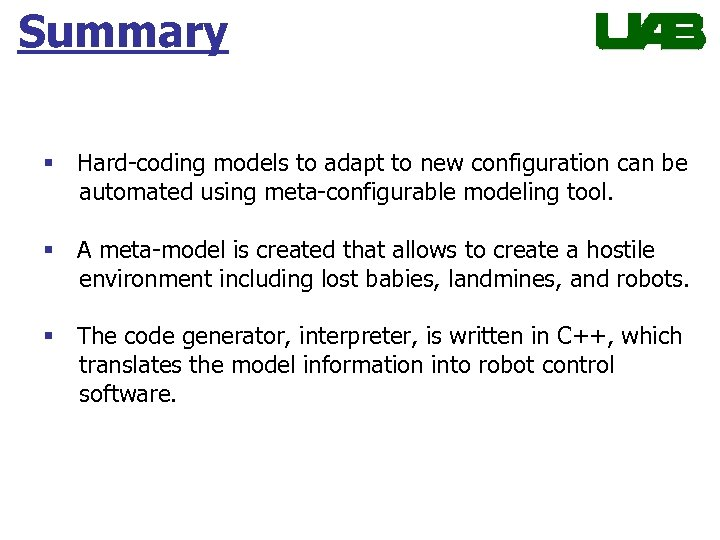Summary § Hard-coding models to adapt to new configuration can be automated using meta-configurable modeling tool. § A meta-model is created that allows to create a hostile environment including lost babies, landmines, and robots. § The code generator, interpreter, is written in C++, which translates the model information into robot control software.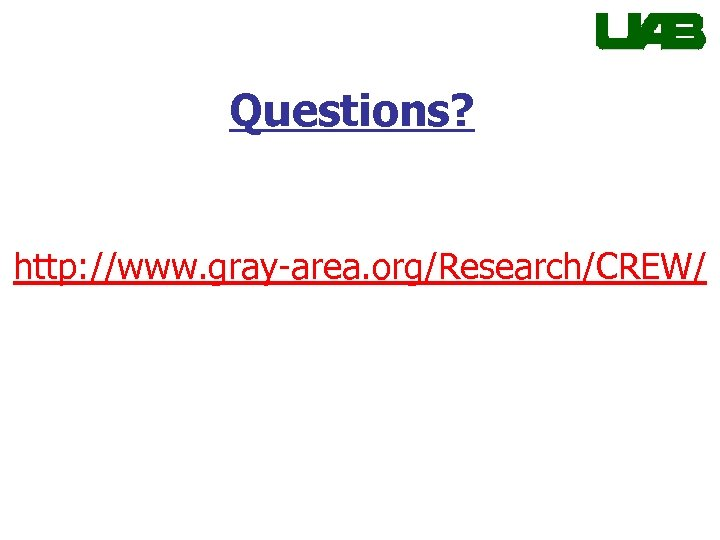Questions? http: //www. gray-area. org/Research/CREW/